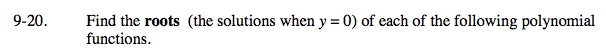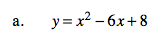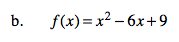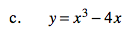Home > A2C > Chapter 9 > Lesson 9.1.1 > Problem9-20

9-20.
1. Find the roots (the solutions when y = 0) of each of the following polynomial functions. Homework Help ✎

1. y = x2 − 6x + 8

2. f(x) = x2 − 6x + 9

3. y = x3 − 4xFactor the right side and use the Zero Product Property.

x = 2, x = 4See part (a).

x = 3See part (a).

x is a common factor.